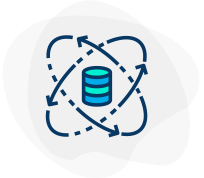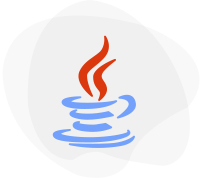# Prerequisites For Machine Learning

### WHAT ARE THE PREREQUISITES FOR MACHINE LEARNING?

Machine learning is a part of Artificial learning and includes the study of algorithms and statistical methods used by computer systems. A specific task is performed in machine learning with the help of patterns and interference of data.

Machine learning is primarily used to reduce manual tasks and automate processes with no human intervention or assistance. It is designed to adapt and adjust to actions accordingly.

For fresher looking to make a career in Machine learning, it is necessary for them to undergo a relevant course for certification in machine learning. But before actually opting for a course they should have the basic knowledge of the necessary skills they need to possess to train themselves in machine learning.

In order to learn Machine learning, it is necessary to have basic knowledge of mathematical concepts.

PREREQUISITES FOR MACHINE LEARNING AND AI

The following basic concepts are necessary for Machine learning:

• Basic mathematics
• Statistics
• Probability
• Linear algebra
• Data modeling
• Calculus
• Programming languages

Now let us understand each and every concept in detail and know why it is a prerequisite for machine learning.

Basic mathematics : Calculus and algebra are the two important aspects of maths that are important to understanding the implementation of algorithms using the given tools. The usefulness and their in-depth knowledge depend upon the type of project users are dealing with. A fresher learning machine learning needs to build algorithms on its own. For such situations having complete knowledge of calculus and algebra is of great help.
Statistics : Statistics is the primary prerequisite for machine learning. The techniques and concepts of machine learning are highly dependent on statistical concepts and theories. It is used to draw a logical conclusion from the huge amount of data available in the database. A person who needs to be trained in machine learning should have the knowledge of working on the concepts of statistics like regression, distribution, and hypothesis testing. The fundamental concepts of statistics used for machine learning are

• Bayes theorem
• Axioms
• Random variables
• Variance and expectation
• Combinatorics
• Conditional and joint distributions

Probability : Probability measures the uncertainty of the event. The higher the probability of an event the more are the chances of its occurrence. Probability is used in machine learning to predict the likelihood of future events with incomplete information. Probability in machine learning helps to design model elements of uncertainty that include designing a transaction process or predicting the risk involved in a business process. The common concepts of probability used in machine learning include:

• Regression with maximum
• Maximum likelihood estimation
• Joint, Marginal, and conditional probability
• Probability distributions (continuous, discrete)
• Density estimation
• Likelihood of Bayes theorem

Linear Algebra : Linear algebra performs operations or transforms the dataset in machine learning. It basically deals with vector operations, matrices, and linear transformations. Linear algebra is an integral part of machine learning as it helps the algorithms to run on a huge number of datasets with multi-dimensional processes. The various concepts of linear algebra that are widely used in machine learning are:

• Optimize the data in machine learning.
• It is used in loss functions, Matrix Operations, covariance matrices, regularisation, Singular Value Decomposition (SVD), and support vector machine classification.
• It is also used for performing Principal Component Analysis for dimensionality reduction.
• It plays a special role in neural networks and the data science field.

Data modeling : It is the process of estimating the structure of a data set. It helps in finding patterns or variations within the set. A major chunk of Machine Learning is usually built on predictive modeling, and therefore with Data Modelling, you would know how to predict different properties of the data. Sometimes, iterative learning algorithms result in magnifying the errors in the set and the model, therefore knowledge of data modeling becomes necessary.

Calculus : Calculus plays an integral part in machine learning. Having a basic knowledge of the concepts is sufficient. Multivariate calculus is used to solve optimization problems in machine learning. Optimization in machine learning is the process of getting the best parameters. Calculus is primarily used to build a model of machine learning. It is an essential component of the algorithm because data sets with multiple features are used to build learning models.

Programming languages : Machine learning algorithms are put into effect with code. Having a basic knowledge of programming is helpful for machine learning. It is necessary to pick at least one programming language to understand the internal mechanisms of machine learning.
Some of the popularly used programming languages in Machine learning are:

Python: The easy syntax, built-in functions, and package support make python a popular programming language.

There are various aspects of Python that are helpful for Machine learning. A few important aspects include:

• Mathematical operations are handled by NumPy
• Deep Learning through TensorFlow
• PyTorch package
• OpenCV and Dlib
• File operations are done by Pandas
• Data Visualisation and more are done through Matplotlib

R: Apart from Python R is another programming language that is widely used for Machine Learning. R programming consists of reliable library support and graphs. This programming language supports the following key packages:

• For regression and classification-based operations, Kernlab and Caret are used
• DataExplorer is used for data exploration
• R part and SuperML for machine learning
• Mlr3 for machine learning workflows
• Plotly and ggplot for data visualization

C++: C++ programming language is mainly used for developing games and complex programming. It helps in logic building and is also used for building libraries.
The key aspects of C++ programming that are helpful for machine learning include:

• Microsoft Cognitive Toolkit (CNTK) and TensorFlow
• Shogun and mlpack
• OpenCV
• OpenNN, FANN, and DyNet

These are the prerequisites for Machine learning. The most popular programming languages used widely by beginners and professionals are Python and R.

Freshers and professionals who are looking to make a career in machine learning should have complete knowledge of these prerequisites. To sum up, machine learning requires statistics, calculus, probability, linear algebra, and knowledge of programming.

## Related Category CoursesAWS DevOps Professional Engineer Course T…

Cloud Computing

AWS DevOps Professional Engineer Course T…
Key FeaturesWhat will I learn?
• 24 Hours of Learning
• 2 Hours of Assignments
• 100% Job AssistanceData Science with Python Training Course …

Artificial Intelligence • Programming & Frameworks

Data Science with Python Training Course …
Key FeaturesWhat will I learn?
• 24 Hours of Learning
• 2 Hours of Assignments
• 100% Job AssistanceJava Course Training Online

Programming & Frameworks

Java Course Training Online
Key FeaturesWhat will I learn?
• 24 Hours of Learning
• 2 Hours of Assignments
• 100% Job AssistancePERL Training Course Online

Programming & Frameworks

PERL Training Course Online
Key FeaturesWhat will I learn?
• 24 Hours of Learning
• 2 Hours of Assignments
• 100% Job AssistanceBigdata • Programming & Frameworks • Machine learning

Key FeaturesWhat will I learn?
• 24 Hours of Learning
• 2 Hours of Assignments
• 100% Job Assistance

Create Account